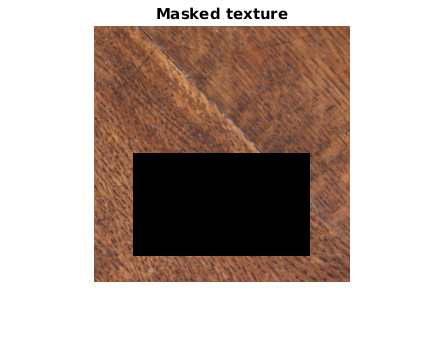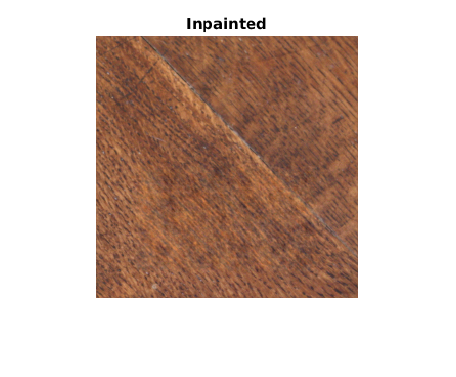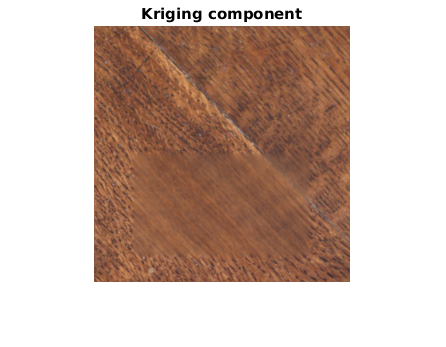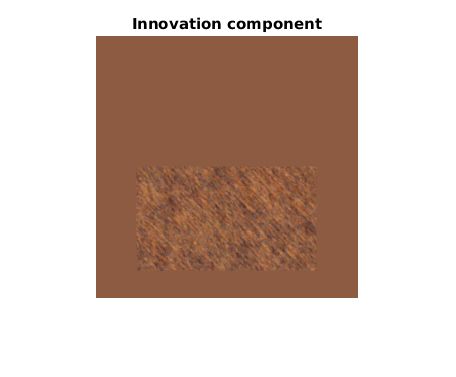Gaussian Texture Inpainting Tutorial

This tutorial can be executed in the Matlab console, provided the archive gaussian_inpainting_matlab.zip has been extracted in the current path.

close all
clear all

in = 'inpainting_input/';

rng(3); % initialize random seed

name = 'wood1008_sq_a_reduced';
[M,N,C] = size(u);
meanu = mean(mean(u,2));

figure
imshow(u)
title('Original')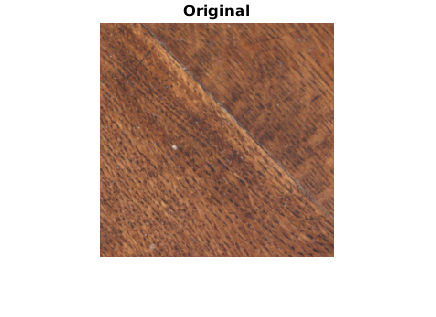x1 = 40; y1 = 128;
x2 = 216; y2 = 230;
indm = zeros(M,N,C);
indm(y1:y2,x1:x2,:) = 1;

figure
imshow(indm)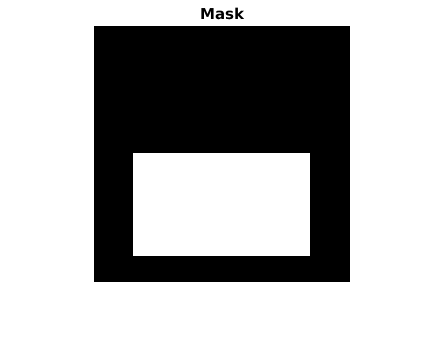Compute the Conditioning points on the mask border

indc = get_conditioning_points(indm,3);

figure
imshow(double(indc))
title('Conditioning points')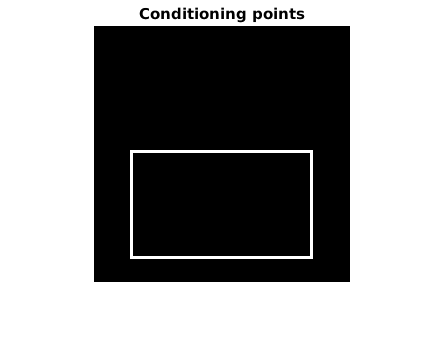Estimate a Gaussian model outside the mask

xo1 = 1; yo1 = 1;
xo2 = N; yo2 = y1-1;
uw = draw_rectangle(u,xo1,xo2,yo1,yo2,2);

figure
imshow(uw.*(1-indm))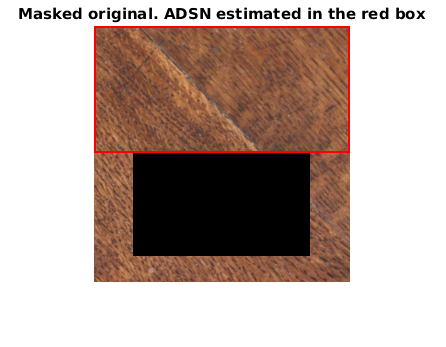Check the texture model

figure
imshow(z)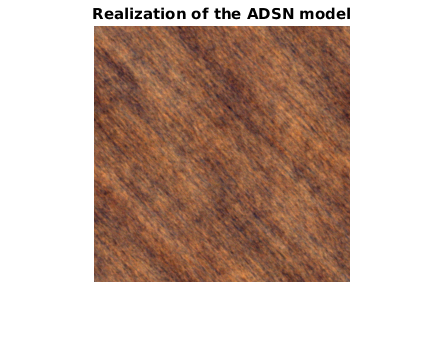Inpaint by Gaussian conditional simulation

[v,kc,innov] = kriging_inpainting(u.*(1-indm),m,t,indm,indc);

figure
imshow(u.*(1-indm));
drawnow

figure
imshow(v);
title('Inpainted')
drawnow

figure
imshow(kc);
title('Kriging component')
drawnow

figure
imshow(innov);
title('Innovation component')
drawnow
Time to solve the linear system of size 5148 x 5148: 1.727